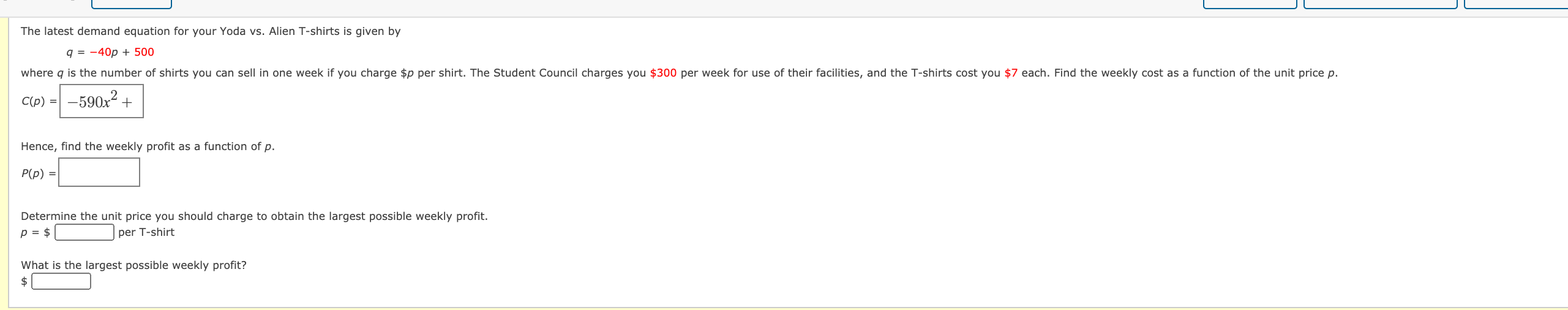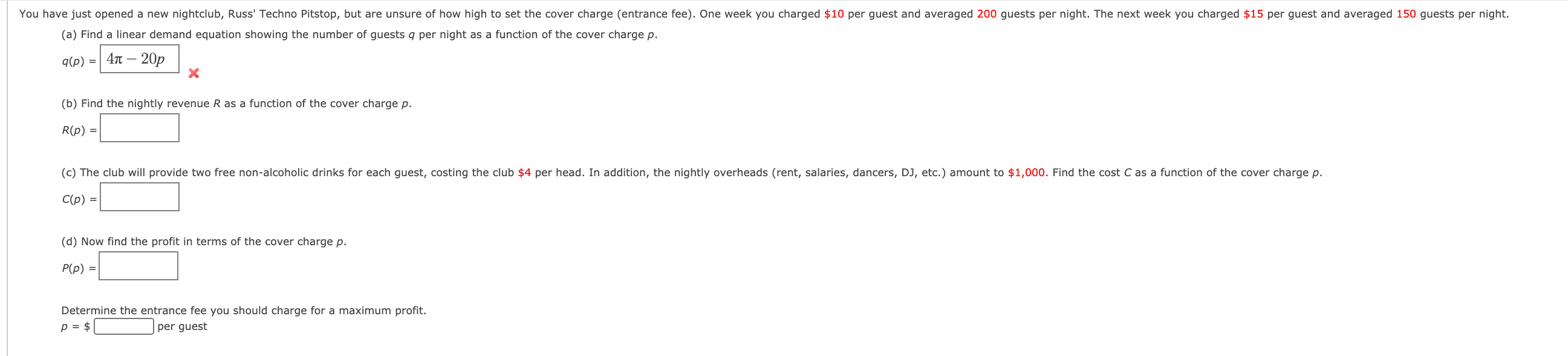# Question Solved1 AnswerThe latest demand equation for your Yoda vs. Alien T-shirts is given by q = -40p + 500 where q is the number of shirts you can sell in one week if you charge \$p per shirt. The Student Council charges you \$300 per week for use of their facilities, and the T-shirts cost you \$7 each. Find the weekly cost as a function of the unit price p. C(p) = -590x2 + Hence, find the weekly profit as a function of p. P(p) = Determine the unit price you should charge to obtain the largest possible weekly profit. p = \$ per T-shirt What is the largest possible weekly profit? \$ You have just opened a new nightclub, Russ' Techno Pitstop, but are unsure of how high to set the cover charge (entrance fee). One week you charged \$10 per guest and averaged 200 guests per night. The next week you charged \$15 per guest and averaged 150 guests per night. (a) Find a linear demand equation showing the number of guests q per night as a function of the cover charge p. – 20p 9(p) = 41 - (b) Find the nightly revenue R as a function of the cover charge p. R(p) = (c) The club will provide two free non-alcoholic drinks for each guest, costing the club \$4 per head. In addition, the nightly overheads (rent, salaries, dancers, DJ, etc.) amount to \$1,000. Find the cost C as a function of the cover charge p. C(p) = (d) Now find the profit in terms of the cover charge p. P(p) = Determine the entrance fee you should charge for a maximum profit. p = \$ per guestTranscribed Image Text: The latest demand equation for your Yoda vs. Alien T-shirts is given by q = -40p + 500 where q is the number of shirts you can sell in one week if you charge \$p per shirt. The Student Council charges you \$300 per week for use of their facilities, and the T-shirts cost you \$7 each. Find the weekly cost as a function of the unit price p. C(p) = -590x2 + Hence, find the weekly profit as a function of p. P(p) = Determine the unit price you should charge to obtain the largest possible weekly profit. p = \$ per T-shirt What is the largest possible weekly profit? \$ You have just opened a new nightclub, Russ' Techno Pitstop, but are unsure of how high to set the cover charge (entrance fee). One week you charged \$10 per guest and averaged 200 guests per night. The next week you charged \$15 per guest and averaged 150 guests per night. (a) Find a linear demand equation showing the number of guests q per night as a function of the cover charge p. – 20p 9(p) = 41 - (b) Find the nightly revenue R as a function of the cover charge p. R(p) = (c) The club will provide two free non-alcoholic drinks for each guest, costing the club \$4 per head. In addition, the nightly overheads (rent, salaries, dancers, DJ, etc.) amount to \$1,000. Find the cost C as a function of the cover charge p. C(p) = (d) Now find the profit in terms of the cover charge p. P(p) = Determine the entrance fee you should charge for a maximum profit. p = \$ per guest
More
Transcribed Image Text: The latest demand equation for your Yoda vs. Alien T-shirts is given by q = -40p + 500 where q is the number of shirts you can sell in one week if you charge \$p per shirt. The Student Council charges you \$300 per week for use of their facilities, and the T-shirts cost you \$7 each. Find the weekly cost as a function of the unit price p. C(p) = -590x2 + Hence, find the weekly profit as a function of p. P(p) = Determine the unit price you should charge to obtain the largest possible weekly profit. p = \$ per T-shirt What is the largest possible weekly profit? \$ You have just opened a new nightclub, Russ' Techno Pitstop, but are unsure of how high to set the cover charge (entrance fee). One week you charged \$10 per guest and averaged 200 guests per night. The next week you charged \$15 per guest and averaged 150 guests per night. (a) Find a linear demand equation showing the number of guests q per night as a function of the cover charge p. – 20p 9(p) = 41 - (b) Find the nightly revenue R as a function of the cover charge p. R(p) = (c) The club will provide two free non-alcoholic drinks for each guest, costing the club \$4 per head. In addition, the nightly overheads (rent, salaries, dancers, DJ, etc.) amount to \$1,000. Find the cost C as a function of the cover charge p. C(p) = (d) Now find the profit in terms of the cover charge p. P(p) = Determine the entrance fee you should charge for a maximum profit. p = \$ per guest